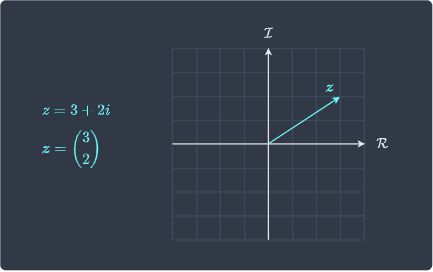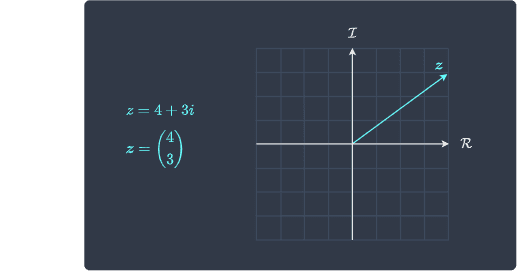search
Search
Unlock 100+ guides
search toc
close
account_circle
Profile
exit_to_app
Sign out
search
keyboard_voice
close
Searching Tips
Search for a recipe:
"Creating a table in MySQL"
Search for an API documentation: "@append"
Search for code: "!dataframe"
Apply a tag filter: "#python"
Useful Shortcuts
/ to open search panel
Esc to close search panel
to navigate between search results
d to clear all current filters
Enter to expand content previewDoc SearchCode Search BetaSORRY NOTHING FOUND!
mic
Start speaking...Voice search is only supported in Safari and Chrome.
Shrink
Navigate to
check_circle
Mark as learned
thumb_up
1
thumb_down
0
chat_bubble_outline
0
Comment
auto_stories Bi-column layout
settings

# Introduction to complex numbers

schedule Aug 12, 2023
Last updated
local_offer
Tags
mode_heat
Master the mathematics behind data science with 100+ top-tier guides
Start your free 7-days trial now!

# Motivation behind complex numbers

Suppose we wanted to solve the equation:

$$\begin{equation}\label{eq:ZvlzW1j1xNtuGdJrjiE} x^2=-1 \end{equation}$$

Clearly, squaring any two real numbers will give us a positive value, which means that this equation is not solvable using real numbers. However, instead of leaving the equation unsolved, mathematicians have come up with a way to express the solution using so-called complex numbers.

Let's go ahead and take the square root of both sides of \eqref{eq:ZvlzW1j1xNtuGdJrjiE} to get:

$$\begin{equation}\label{eq:O3x7ZSRvNzraAw1ynGS} x=\pm\sqrt{-1} \end{equation}$$

Again, it does not make sense to take the square root of a negative number. At the heart of complex numbers is the imaginary number $i$ defined below:

$$\begin{equation}\label{eq:rgn4V6FtYzgAOontgL0} i=\sqrt{-1} \end{equation}$$

The solution \eqref{eq:O3x7ZSRvNzraAw1ynGS} can now be expressed as:

$$x=\pm{i}$$

The reason we somewhat forcefully solve these equations using complex numbers is to understand more about the nature of the equation instead of marking it as unsolvable. As we shall explore in the latter chapters, complex numbers are required to prove important theorems such as the fundamental theorem of algebra and the central limit theorem.

Definition.

# Complex numbers

If $a$ and $b$ are real numbers while $i=\sqrt{-1}$, then any number of the form $a+bi$ is called a complex number.

• we often denote a complex number as $z$.

• some textbooks define the imaginary number as $i^2=-1$.

Example.

## Some complex number

The following are all examples of complex numbers:

\begin{align*} z_1&=4+6i\\ z_2&=3\\ z_3&=7i\\ z_4&=\pi \end{align*}

Notice how $z_2=3$ which appears to be just a real number is also a complex number. This is because $z_2$ can be written as $z_2=3+0i$. In this way, all real numbers including $\pi$ can be written as a complex number. Therefore, real numbers are considered to be a subset of complex numbers.

Definition.

# Set of all complex numbers

Just like how $\mathbb{R}$ is the set of all real numbers, we can define $\mathbb{C}$ as the set of all complex numbers. Since real numbers are a subset of complex numbers, we have that $\mathbb{R}\subset\mathbb{C}$.

Definition.

# Real and imaginary part of complex numbers

If $z=a+bi$ is any complex number, then:

• $a$ is known as the real part of $z$, denoted by $\mathcal{Re}(z)$.

• $b$ is known as the imaginary part of $z$, denoted by $\mathcal{Im}(z)$.

Example.

## Finding the real and imaginary part of a complex number

Consider the following complex numbers:

• $z_1=2+3i$.

• $z_2=5$.

Find the real parts and imaginary parts of $z_1$ and $z_2$.

Solution. Let's first tackle $z_1$. The real part of $z_1$ is $2$ and the imaginary part of $z_1$ is $3$, that is:

\begin{align*} \mathcal{Re}(z_1)&=2\\ \mathcal{Im}(z_1)&=3\\ \end{align*}

Next, the real and imaginary parts of $z_2$ are:

\begin{align*} \mathcal{Re}(z_2)&=5\\ \mathcal{Im}(z_2)&=0\\ \end{align*}
Definition.

## Purely real and purely imaginary complex numbers

Suppose we have a complex number $z=a+bi$.

• if $a\ne0$ and $b=0$, then the complex number is called purely real.

• if $a=0$ and $b\ne0$, then the complex number is called purely imaginary.

# Arithmetic operations of complex numbers

Just like for real numbers, we can also add, subtract, multiply and divide between complex numbers.

$$(a+bi)+(c+di)=(a+c)+(b+d)i$$

It is always a good idea to explicitly split the complex number into its real and imaginary parts as we did here.

## Subtraction

Subtracting a complex number from another complex number:

$$(a+bi)-(c+di)=(a-c)+(b-d)i$$

## Multiplication

Multiplying two complex numbers:

\begin{align*} (a+bi)(c+di)&= ac+adi+bci+bdi^2\\ &=ac-bd+(ad+bc)i \end{align*}

Here, we used the fact that $i^2=-1$.

## Division

Dividing two complex numbers is slightly tricky. We multiply both the numerator and denominator by $c-di$ so that the imaginary part disappears from the denominator:

\begin{align*} \frac{a+bi}{c+di}&= \Big(\frac{a+bi}{c+di}\Big) \Big(\frac{c-di}{c-di}\Big)\\ &=\frac{ac-adi+bci-bdi^2}{c^2-cdi+cdi-d^2i^2}\\ &=\frac{ac-adi+bci+bd}{c^2+d^2}\\ &=\frac{ac+bd+(bc-ad)i}{c^2+d^2}\\ \end{align*}
Example.

## Performing arithmetic operations on complex numbers

Consider the following complex numbers:

\begin{align*} z_1&=3+2i\\ z_2&=1+i\\ \end{align*}

Compute the following:

1. $z_1+z_2$.

2. $z_1-z_2$.

3. $z_1z_2$.

4. $z_1/z_2$.

\begin{align*} z_1+z_2&=(3+2i)+(1+i)\\ &=4+3i \end{align*}

2. Subtraction is:

\begin{align*} z_1-z_2&=(3+2i)-(1+i)\\ &=2+i \end{align*}

3. Multiplication is:

\begin{align*} z_1z_2 &=(3+2i)(1+i)\\ &=3+3i+2i+2i^2\\ &=3+5i-2\\ &=1+5i\\ \end{align*}

4. Division is:

\begin{align*} \frac{z_1}{z_2}&= \frac{3+2i}{1+i}\\ &=\frac{3+2i}{1+i}\Big(\frac{1-i}{1-i}\Big)\\ &=\frac{3-i-2i^2}{1^2-i^2}\\ &=\frac{3-i+2}{1+1}\\ &=\frac{5-i}{2}\\ &=\frac{5}{2}-\frac{1}{2}i \end{align*}

# Properties of arithmetic operations of complex numbers

Theorem.

## Commutative property of multiplication of complex numbers

If $z_1$ and $z_2$ are complex numbers, then:

$$z_1z_2=z_2z_1$$

This means that we can perform the multiplication in either order.

Proof. Let $z_1=a+b_i$ and $z_2=c+d_i$. Now, the product $z_1z_2$ is:

\begin{align*} z_1z_2&= (a+bi)(c+d_i)\\ &=ac+adi+bci+bdi^2\\ &=ca+cbi+dai+dbi^2\\ &=(c+di)(a+bi)\\ &=z_2z_1 \end{align*}

This completes the proof.

Theorem.

## Associativity property of multiplication of complex numbers

If $z_1$, $z_2$ and $z_3$ are complex numbers, then:

$$(z_1z_2)z_3 =z_1(z_2z_3)$$

Proof. We define complex numbers $z_1$, $z_2$ and $z_3$ like so:

\begin{align*} z_1&=a_1+b_1i\\ z_2&=a_2+b_2i\\ z_3&=a_3+b_3i\\ \end{align*}

We start from the left-hand side:

\begin{align*} (z_1z_2)z_3 &=\big((a_1+b_1i)(a_2+b_2i)\big)(a_3+b_3i)\\ &=(a_1a_2+a_1b_2i+a_2b_1i+b_1b_2i^2\big)(a_3+b_3i)\\ &=a_1a_2a_3+a_1b_2a_3i+a_2b_1a_3i+a_3b_1b_2i^2+ a_1a_2b_3+a_1b_2b_3i+a_2b_1b_3i+b_1b_2b_3i^2\\ &={\color{blue}a_1}a_2a_3+{\color{blue}a_1}a_2b_3+{\color{blue}a_1}b_2a_3i+{\color{blue}a_1}b_2b_3i+ {\color{red}b_1}a_2a_3i+{\color{red}b_1}a_2b_3i+{\color{red}b_1}b_2a_3i^2+{\color{red}b_1}b_2b_3i^2\\ &=({\color{blue}a_1}+{\color{red}b_1}i)(a_2a_3+a_2b_3+b_2a_3i+b_2b_3i)\\ &=({\color{blue}a_1}+{\color{red}b_1}i)\big((a_2+b_2i)(a_3+b_3i)\big)\\ &=z_1(z_2z_3) \end{align*}

This completes the proof.

# Visualizing complex numbers using the Argand plane

We can plot complex numbers on a so-called complex plane or the Argand plane. For instance, the complex number $z=3+2i$ can be visualized as:The key difference between the traditional Cartesian plane and the Argand plane is what the axis lines represent. In Cartesian plane:

• the horizontal axis represents the $x$-axis.

• the vertical axis represents the $y$-axis.

In contrast, for an Argand plane:

• the horizontal axis represents the real part of the complex number.

• the vertical axis represents the imaginary part of the complex number.

In fact, there is a one-to-map mapping between the Cartesian plane and the Argand plane. For instance, the point $(3,2)$ in the Cartesian plane corresponds to the complex number $z=3+2i$ in the Argand plane.

Definition.

# Modulus of a complex number

Let $z$ be a complex number defined as $z=a+bi$. The modulus of $z$ is denoted as $\vert{z}\vert$ and is defined as:

$$\vert{z}\vert=\sqrt{a^2+b^2}$$

Note that the modulus is always a real number since $a^2+b^2$ is always non-negative.

Discussion. The reason we use the absolute value symbol to indicate the modulus is that if $b=0$, then:

$$\vert{z}\vert=\sqrt{a^2}=\vert{a}\vert$$

Here, the right-hand side represents the absolute value of the real number $a$. This means that if the imaginary part of the complex number is zero, then the modulus can be interpreted as the absolute value.

Example.

## Finding the modulus of a complex number

Let $z=4+3i$. Find the modulus of $z$.

Solution. The modulus of $z$ is:

\begin{align*} \vert{z}\vert&= \sqrt{4^2+3^2}\\ &=5 \end{align*}

Let's also visualize $z$ on the Argand plane:Here, the modulus $\vert{z}\vert$ represents the length of this vector.

thumb_up
thumb_down
Comment
Citation
Ask a question or leave a feedback...
thumb_up
1
thumb_down
0
chat_bubble_outline
0
settings
Enjoy our search
Hit / to insta-search docs and recipes!